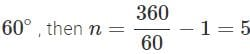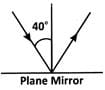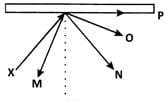## 20 Questions MCQ Test General Science(Prelims) by IRS Divey Sethi | Olympiad Test: Light

Description
Attempt Olympiad Test: Light | 20 questions in 20 minutes | Mock test for UPSC preparation | Free important questions MCQ to study General Science(Prelims) by IRS Divey Sethi for UPSC Exam | Download free PDF with solutions
QUESTION: 1

### X is a surface that cannot produce clear images. What is X?

Solution:

When light is incident on a rough surface, irregular reflection takes place, hence the image formed is not clear.

QUESTION: 2

### With what is glass coated in order to convert it into a mirror?

Solution:

Glass is coated with silver.

QUESTION: 3

### What makes objects visible?

Solution:

An object is visible only when reflected light from the object reaches the retina of our eyes.

QUESTION: 4

What is the phenomenon of light bouncing back into the same medium called?

Solution:

The phenomenon of light in which, light is reflected or bounced back into the same medium is called reflection.

QUESTION: 5

What is the perpendicular drawn at any point on a mirror called?

Solution:

The perpendicular drawn to the reflecting surface of a mirror at the point of incidence is called 'normal'. The point on the reflecting surface where the light ray is incident is called the point of incidence.

QUESTION: 6

How many images are obtained when plane mirrors are arranged parallel to each other?

Solution:

When two mirrors are kept parallel to each other, an infinite number of images can be seen.

QUESTION: 7

If the angle of incidence is 80o, what will be the angle of reflection?

Solution:

The angle of incidence = the angle of reflection. So, the angle of reflection is 80o.

QUESTION: 8

Which of the following is used by E.N.T. doctors?

Solution:

A concave mirror is used by E.N.T. doctors to get a magnified image of internal organs like ear, nose and throat.

QUESTION: 9

A When the angle between two plane mirrors is 60, how many images will be formed by the mirrors?

Solution:

If two plane mirrors are inclined at an angle ofimages are formed.

QUESTION: 10

If the angle of incidence is 50o, then calculate the angle between the incident ray and the reflected ray.

Solution:

In reflection of light, ∠i = 50oand ∠i = ∠r = the angle between incident ray and reflected ray = 50° + 50° = 100°

QUESTION: 11

In our houses, which of the following is used for looking at ourselves?

Solution:

We use plane mirrors to see ourselves, because the image formed by a plane mirror is virtual, erect and of the same size.

QUESTION: 12

Which of the following statements is true?

Solution:

When a light ray is reflected from the reflecting surface, the incident ray, the reflected ray and the normal lie in the same plane. The angle of incidence = The angle of reflection.

QUESTION: 13

What happens in lateral inversion?

Solution:

The image formed by the plane mirror is laterally inverted i.e., the right side of the object appears on the left side of the image and left side of the object appears on the right side of the image.

QUESTION: 14

In a periscope, how are the reflecting mirrors arranged?

Solution:

Explanation:- A periscope is an optical instrument used to view object which is not in direct sight of human eye. It works by using the Laws of Reflection and are widely used in submarines to navigate under water.

It works by using two mirrors placed at angle of 45 degree with each other. When light falls on one mirror, it bounces to the other mirror and then reaches the human eye.

A simple periscope only uses mirrors but complex periscope uses prisms instead of mirror. These periscopes are used in the field of medicine particularly in cystoscopy and endoscopy.

QUESTION: 15

What is the nature of image formed on the retina of human eye of an object?

Solution:

The human eye serves as a convex lens. It forms real, inverted and diminished image of an object.

QUESTION: 16

Identify the value of persistence of vision.

Solution:

Persistence of vision is a phenomenon where the brain continues to sense the image even after the object has been removed. This lasts for 1/16th of a second.

QUESTION: 17

The characteristics of an eye disease are given below.
(i) Eye sight becomes foggy
(ii) Eye lens becomes cloudy
(iii) There is a loss of vision

In which of the following are the above characteristics observed?

Solution:

Cataract occurs in old age due to the given characteristics and can be cured by introducing a new artificial lens.

QUESTION: 18

Which of the following is the requirement of nocturnal animals like owl an bat?

Solution:

The nocturnal animals need more light to see at night. The large cornea and pupil allow more light to enter into their eyes. They also have retina with large number of rods.

QUESTION: 19

Look at the given figure.Find the angle between the incident ray and the reflected ray.

Solution:

∠i = 40o(given) ∠i = ∠r = 40o
The angle between the incident ray and the reflected ray =∠i +∠r = 40o+40o = 80o

QUESTION: 20

The diagram shows the path of a light ray X, directed at a plane mirror.Which of the following is the correct reflected ray?

Solution:

We know that for a reflected ray, the angle that the incident ray makes with the line perpendicular to the surface is equal to the angle made by the reflected ray with this perpendicular line. N is the correct reflected ray of X.Use Code STAYHOME200 and get INR 200 additional OFF Use Coupon Code

### How to Prepare for UPSC

Read our guide to prepare for UPSC which is created by Toppers & the best Teachers